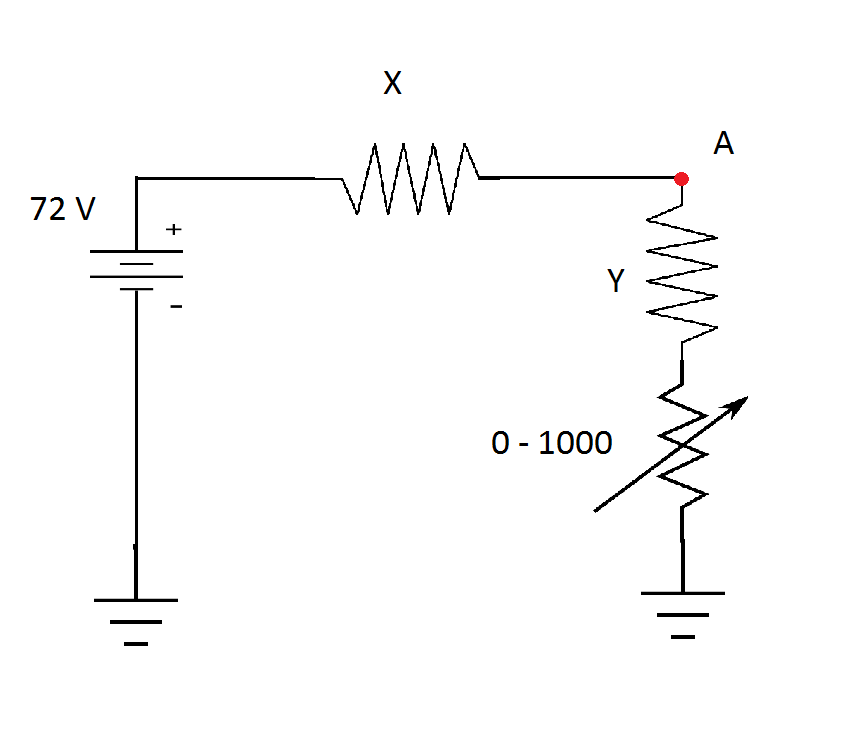# Is this simple ratio and proportion?For the given circuit above, resistors $X$ and $Y$ have fixed values, and the range of resistance of the variable resistor is 0 to 1000 ohms. If the variable resistor is set at $0\:\Omega$, $V_A = 27\: V$, and $V_A = 52\: V$ when it is set to $1000 \:\Omega$. Determine at what resistance (in ohms) must the variable resistor be set such that the output voltage measured between $A$ and ground is $42 \: V$.

×